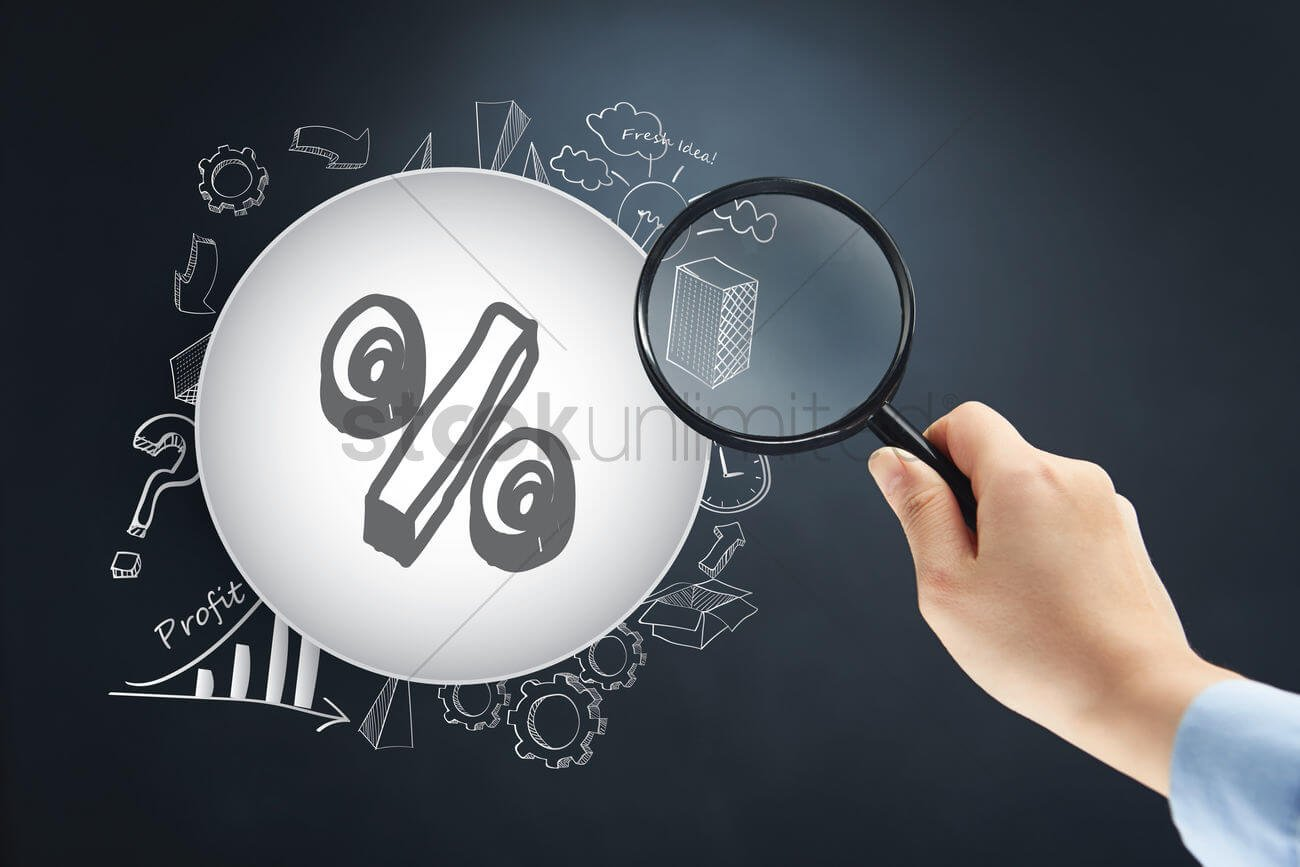# THE BASIC IDEA OF PERCENTAGE AND WHY IS IT USEFUL?

Introduction: The symbol of percentage is so common that you see it every day. Whenever you visit a mall you will see that the shopping companies give discounts. These discounts do not have any unit but a percentage symbol. It is something that allows you to find the ratio of a substance from 100. It gives the students a standard reference value for something. If you say that you want to buy 5 parts of the cake, the shopkeeper will not understand. It is because the cake as a whole has no fixed value. When you say that you want 5 percent of the cake, you compare the cake with the value 100.

## How to calculate it?

Calculating the percentage is not a big deal if you understand the logic. You have to find the fraction of the item that is present. In every percentage sum, you need to calculate the fraction first. The examiner will give you certain situations that you have to understand. Take an example where the question says that 6 people have come to class today. The class has a total number of students that is equal to 10. In this case, you need to form a fraction by dividing 6 by 10. You will get a decimal value of 0.6. The final step to get the percentage value is to multiply it by 100. Then you will understand that only 60 percent of students came to the class.

## How to find percentage change?

This is another vital application where the percentage is used. Not only can you find a fixed value but also compare the changes. It is something that statisticians and salespeople use a lot. You can understand the concept from a basic example. Suppose there is a cloth with an M.R.P of 100 rupees. After a few days, the market price may rise to the value of 150. To calculate the percentage change, first, subtract the value.

You have to keep the sign negative during the subtraction. After that, you need to divide the result with the original price. Many kids get the wrong answer as they divide the change with the changed price. But that is not the aim of the solution in this chapter. Dividing 50 with 100 you will get the fraction 0.5. Similarly, multiply with 100 and you will get 50 percent as the answer.

## What is the profit percentage?

Percentage change is also required in the chapter on Profit and Loss. To calculate profit the division of selling and cost price is essential. After that, the question may ask you to find out the total percentage of the profit. In the field of business, this term is used a lot. To approach this kind of sum you need the same thinking.

Whenever you need to find the percentage of change the original price is a must. In the pIDEA OF PERCENTAGErofit and loss sums the cost price will always be the original price. This is the price at which the manufacturer gave the item to the shop owner. Thus you need to divide the profit with cost price to get a fraction. Multiplying with a hundred is a step that you need to keep constant throughout. Similarly, in a few sums, they may give the profit percentage directly. In that situation, you need to multiply the cost price by a fraction of 100. This will give you the exact value in that unit as well. The percentage does not have any unit because it is only a ratio.

The percentage is a topic that people use to describe measurements in real life. Get the link to math online classes from Cuemath, one of the best online platforms to get a proper solution to your questions.